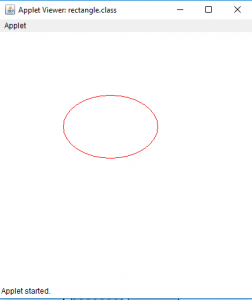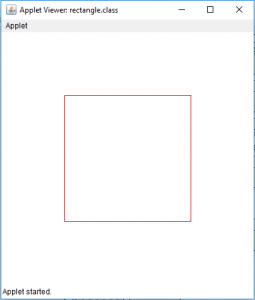# Draw a ellipse and a rectangle in Java Applet

• Difficulty Level : Hard
• Last Updated : 02 Jun, 2022

Java applets are application that can be executed in web browsers or applet viewers . We can draw shapes on the Java applet. In this article we will draw a ellipse on Java applet by two ways . By using the drawOval(int x, int y, int width, int height) or by using mathematical formula (X= A * sin a, Y= B *cos a, where A and B are major and minor axes and a is the angle ) . Similarly, we will draw a rectangle on Java applet by two ways . By using the drawRect(int x, int y, int width, int height) or by drawing four lines joining the edges .

To draw a ellipse in Java Applet

Examples: Let us draw a oval with width 150 and height 100

```Input : x and y coordinates 100, 100 respectively
Width and height 150 and 100 respectively ```

Output :To draw a rectangle in Java Applet

Examples: We will draw a rectangle of height 200 and width 200 and At a position 100,100 on the applet.

```Input : x and y coordinates 100, 100 respectively
Width and height 200 and 200 respectively.```

Output :1. Java Program to draw a ellipse using drawOval(int x, int y, int width, int height)

## Java

 `// java program to draw a ellipse` `// using drawOval function.` `import` `java.awt.*;` `import` `javax.swing.*;`   `public` `class` `ellipse ``extends` `JApplet {`   `    ``public` `void` `init()` `    ``{` `        ``// set size` `        ``setSize(``400``, ``400``);`   `        ``repaint();` `    ``}`   `    ``// paint the applet` `    ``public` `void` `paint(Graphics g)` `    ``{` `        ``// set Color for rectangle` `        ``g.setColor(Color.red);`   `        ``// draw a ellipse` `        ``g.drawOval(``100``, ``100``, ``150``, ``100``);` `    ``}` `}`

Output :2. program to draw a ellipse using drawLine function

## Java

 `// java program to draw a ellipse` `// using drawLine function` `import` `java.awt.*;` `import` `javax.swing.*;`   `public` `class` `ellipse ``extends` `JApplet {`   `    ``public` `void` `init()` `    ``{` `        ``setSize(``300``, ``300``);` `    ``}`   `    ``public` `void` `paint(Graphics g)` `    ``{` `        ``// center of the` `        ``int` `cx, cy;`   `        ``// center of the ellipse` `        ``cx = ``150``;` `        ``cy = ``175``;`   `        ``// major and minor axis` `        ``double` `A = ``75``, B = ``50``, px = ``0``, py = ``0``;`   `        ``// set color` `        ``g.setColor(Color.red);`   `        ``// draw the ellipse` `        ``for` `(``int` `i = ``0``; i <= ``360``; i++) {` `            ``double` `x, y;` `            ``x = A * Math.sin(Math.toRadians(i));` `            ``y = B * Math.cos(Math.toRadians(i));`   `            ``if` `(i != ``0``) {` `                ``// draw a line joining previous and new point .` `                ``g.drawLine((``int``)px + cx, (``int``)py + cy,` `                                ``(``int``)x + cx, (``int``)y + cy);` `            ``}`   `            ``// store the previous points` `            ``px = x;` `            ``py = y;` `        ``}` `    ``}` `}`

Output :Now we will see how to draw a rectangle in a Java Applet We can draw rectangle in java applet by two ways.

1. Draw a rectangle using drawRect(int x, int y, int width, int height)

## Java

 `// Java Program to  Draw a rectangle` `// using drawRect(int x, int y, int width, int height)` `import` `java.awt.*;` `import` `javax.swing.*;`   `public` `class` `rectangle ``extends` `JApplet {`   `    ``public` `void` `init()` `    ``{` `        ``// set size` `        ``setSize(``400``, ``400``);`   `        ``repaint();` `    ``}`   `    ``// paint the applet` `    ``public` `void` `paint(Graphics g)` `    ``{` `        ``// set Color for rectangle` `        ``g.setColor(Color.red);`   `        ``// draw a rectangle` `        ``g.drawRect(``100``, ``100``, ``200``, ``200``);` `    ``}` `}`

Output:2. Draw a rectangle using drawLine(int x, int y, int x1, int y1)

## Java

 `// Java Program  Draw a rectangle` `// using drawLine(int x, int y, int x1, int y1)` `import` `java.awt.*;` `import` `javax.swing.*;`   `public` `class` `rectangle ``extends` `JApplet {`   `    ``public` `void` `init()` `    ``{` `        ``// set size` `        ``setSize(``400``, ``400``);`   `        ``repaint();` `    ``}`   `    ``// paint the applet` `    ``public` `void` `paint(Graphics g)` `    ``{` `        ``// set Color for rectangle` `        ``g.setColor(Color.red);`   `        ``// draw a rectangle by drawing four lines` `        ``g.drawLine(``100``, ``100``, ``100``, ``300``);` `        ``g.drawLine(``100``, ``300``, ``300``, ``300``);` `        ``g.drawLine(``300``, ``300``, ``300``, ``100``);` `        ``g.drawLine(``300``, ``100``, ``100``, ``100``);` `    ``}` `}`

Output :Note : the following programs might not run in an online compiler please use an offline IDE.

My Personal Notes arrow_drop_up
Recommended Articles
Page :